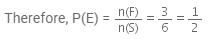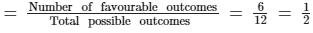Courses

# Test: Classical Approach To Probability

## 15 Questions MCQ Test Mathematics (Maths) Class 10 | Test: Classical Approach To Probability

Description
This mock test of Test: Classical Approach To Probability for Class 10 helps you for every Class 10 entrance exam. This contains 15 Multiple Choice Questions for Class 10 Test: Classical Approach To Probability (mcq) to study with solutions a complete question bank. The solved questions answers in this Test: Classical Approach To Probability quiz give you a good mix of easy questions and tough questions. Class 10 students definitely take this Test: Classical Approach To Probability exercise for a better result in the exam. You can find other Test: Classical Approach To Probability extra questions, long questions & short questions for Class 10 on EduRev as well by searching above.
QUESTION: 1

### If the probability of winning a game is 0.995, then the probability of losing is

Solution:

Let P(winning the game)=0.995

Since the probability of two complimentary event sums to 1 so-

P(not winning the game) + P(winning the game )=1

=P(losing it )=1-P(winning the game )

=1-0.995=0.005

QUESTION: 2

### What is the probability of a sure event?​

Solution:

A sure event is an event, which always happens. For example, it's a sure event to obtain a number between “1”“1” and “6”“6” when rolling an ordinary die.

The probability of a sure event has the value of 11.

QUESTION: 3

### The probability of an event that is certain to happen is

Solution:

The probability of an event is a number describing the chance that the event will happen. An event that is certain to happen has a probability of 1. An event that cannot possibly happen has a probability of zero. If there is a chance that an event will happen, then its probability is between zero and 1.

Examples of Events:

• tossing a coin and it landing on heads
• tossing a coin and it landing on tails
• rolling a '3' on a die
• rolling a number > 4 on a die
• it rains two days in a row
• drawing a card from the suit of clubs
• guessing a certain number between 000 and 999 (lottery)
QUESTION: 4

Which of the following cannot be the probability of an event?​

Solution:

Probability of an event (E) is always greater than or equal to 0.

Also, it is always less than or equal to one.

This implies that the probability of an event cannot be negative or greater than 1.

Therefore, out of these alternatives, −1.5 cannot be a probability of an event.

QUESTION: 5

The probability of getting a prime number in single throw of a dice is:​

Solution:

Given, A dice is thrown once. So,

Total number of outcomes (n) = 6

Number of prime numbers = {2, 3,5}

So, Favorable number of outcomes (m) = 3

Thus, probability of getting a prime number = m/n = 3/6 = 1/2

QUESTION: 6

If a die is thrown once, the probability of getting a prime number is​

Solution:

Possible numbers of events on throwing a dice = 6
Numbers on dice = 1,2,3,4,5 and 6

Prime numbers = 2, 3 and 5
Favourable number of events = 3
Probability that it will be a prime number = 3/6 = 1/2

QUESTION: 7

The probability that a non-leap year selected at random will have 53 Mondays is

Solution:

We know that there are 52 weeks in a year.

There are 7 days in a week

52 weeks will be  to 7*52=364 days.

The remaining 1 day can be any day among Monday, Tuesday,..., Sunday.
Sample space has seven days as options.
So probability of getting 53 Sundays in a non-leap year is 1/7

QUESTION: 8

A card is drawn at random from a pack of 52 playing cards. The probability of getting a face card is

Solution:

Clearly, there are 52 cards, out of which there are 12 face cards.

P (getting a face card) = 12/52 = 3/13.

QUESTION: 9

Which one of the following cannot be the probability of an event?​

Solution:

If the probability is in percentage we divide it by 100
So d) option is 0.05.And we know If an event is impossible its probability is zero. Similarly, if an event is certain to occur, its probability is one. The probability of any event lies in between these values. It is called the range of probability and is denoted as 0 ≤ P (E) ≤ 1.And probability more than one means that favourable outcomes are more than total outcomes which is wrong.

QUESTION: 10

If the probability of winning a game is 0.3, then the probability of losing it is:​

Solution:

Let the probability of losing the game be x
We know that sum of the the sum of probabilities is equal to 1
So
0.3+x=1
x=0.7

QUESTION: 11

If a letter of English alphabet is chosen at random, then the probability that the letter is a consonant is:

Solution:

The probability that the letter is a consonant = (26 - 5)/26 = 21/26.

QUESTION: 12

If a digit is chosen at random from the digits 1, 2, 3, 4, 5, 6, 7, 8, 9 then the probability that it is odd is​

Solution:

Sample Space=1,2,3,4,5,6,7,8,9
Total number of outcomes=9
No of favourable outcomes(odd)=1,3,5,7,9=5
Probability of odd number=No.of favourable outcomes/total number of outcomes=5/9

QUESTION: 13

Two coins are tossed together. The probability of getting head on both the coins is​

Solution:

The sample space for the event is :    (H,H)  (T,H)  (H,T)  (T,T)
Therefore total outcomes= 4

Probability =  1/4

QUESTION: 14

The probability of getting an even number, when a die is thrown once, is:​

Solution:

If we throw a die once, then possible outcomes (s), are
S = { 1, 2, 3, 4, 5, 6 }
⇒    n(E) = 6
(i) Let E be the favourable outcomes of getting an even number, then
E = { 2, 4, 6 }
⇒ n(S) = 3QUESTION: 15

The probability that a randomly chosen number from one to twelve is a divisor of twelve is​

Solution:

We have the numbers from 1 to 12. Total possible outcomes = 12 Now, divisors of 12 : 1, 2, 3, 4, 6 and 12 Number of divisors of 12 = 6 Number of favourable outcomes = 6
Required probability